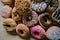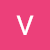# Asymptotic Runtime Complexity — Analyzing Time and SpaceMy definition of Big-O Notation.

In computational theory, the efficiency and performance of an algorithm can be mathematically quantified by comparing its’ arbitrary input size against the runtime. However, an identical algorithm (or even a program) being executed on one computer may not necessarily have the same runtime on another computer. Dependencies such as processor speed, I/O speed, cache size, and etc. are without a doubt contributing factors. As such, the fundamentals behind this theory of analysis is asymptotic in nature.

Asymptotic (asymptote) is defined by their ratio as a given variable approaches infinity.

Take the Golden Ratio (φ) for example. Given its algebraic formula,

`…`